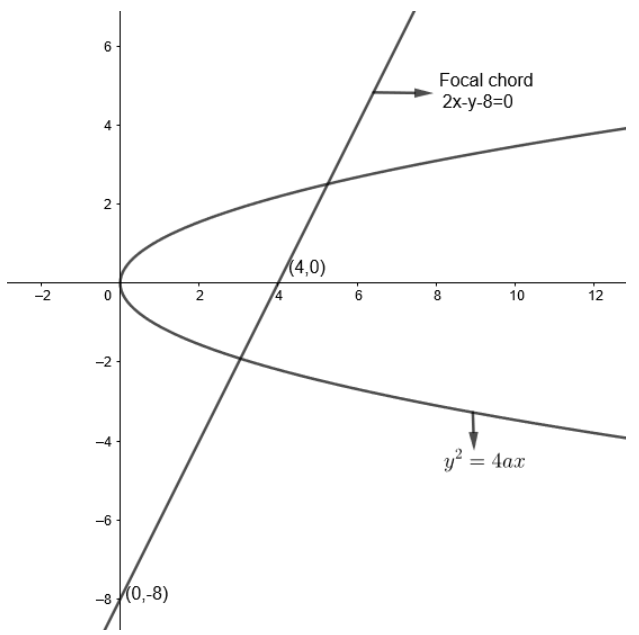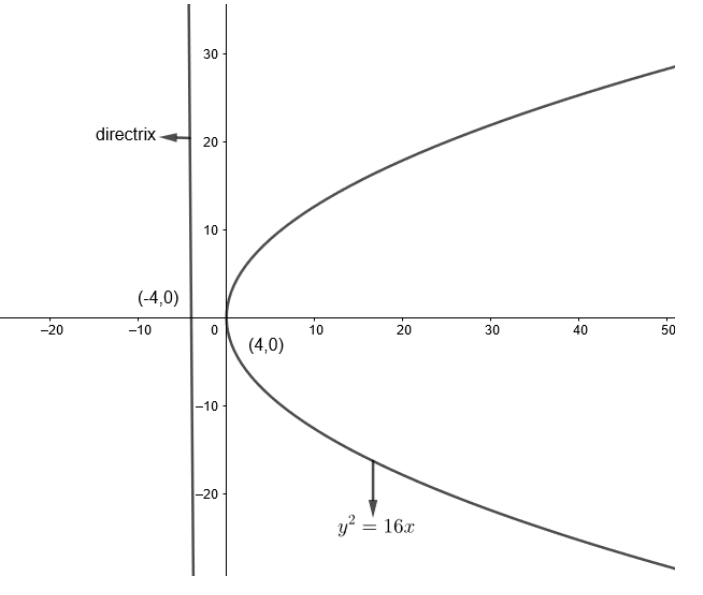QuestionAnswers

# If a focal chord of the parabola ${{y}^{2}}=ax$is $2x-y-8=0$, then the equation of the directrix is:(a) $y+4=0$(b) $x-4=0$(c) $y-4=0$(d) $x+4=0$Verified
158.7k+ views
Hint: Compare the results of general parabola with given parabola to find “$a$”of given parabola.

Given that the focal chord of parabola ${{y}^{2}}=ax$ is $2x-y-8=0$.We know that focal chord is a chord which passes through the focus of parabola.
For standard parabola, ${{y}^{2}}=4ax$.
Focus is at $\left( x,y \right)=\left( \dfrac{4a}{4},0 \right)=\left( a,0 \right)$
Therefore for given parabola, ${{y}^{2}}=ax$
We get, focus at $\left( x,y \right)=\left( \dfrac{a}{4},0 \right)$.
The given focal chord passes through focus.
Therefore, substituting $x=\dfrac{a}{4},y=0$in $2x-y-8=0$
We get, $2\left( \dfrac{a}{4} \right)-\left( 0 \right)-8=0$
$=\dfrac{a}{2}-8=0$
Therefore, we get $a=16$
Hence, we get parabola ${{y}^{2}}=ax$
$\Rightarrow {{y}^{2}}=16x$For general parabola, ${{y}^{2}}=4ax$
Directrix is $x=\dfrac{-4a}{4}$
$\Rightarrow x=-a$
Or $x+a=0$
Therefore, for given parabola
${{y}^{2}}=16x$
We get, directrix $\Rightarrow x=\dfrac{-16}{4}$
$\Rightarrow x=-4$
Or, $x+4=0$
Therefore (d) is the correct option.
Note: As we know that, for standard parabola, focus lies on$x$axis, we can directly find focus by putting
$y=0$in given focal chord which is as follows:
Now, we put $y=0$in equation $2x-y-8=0$.
We get, $2x-\left( 0 \right)-8=0$
$x=\dfrac{8}{2}=4$
Therefore, focus $\left( a,0 \right)$is $\left( 4,0 \right)$.
Also, a directrix could be found by taking a mirror image of focus through the$y$axis which would be
$\left( -4,0 \right)$.
As we know that the directrix is always perpendicular to the $x$ axis and passes through $\left( -4,0 \right)$.
Here, therefore equation of directrix is:
$x=\text{constant}$
And here $\text{constant}=-4$
Therefore, we get equation of directrix as $x=-4$or $x+4=0$
Hence, option (d) is correct